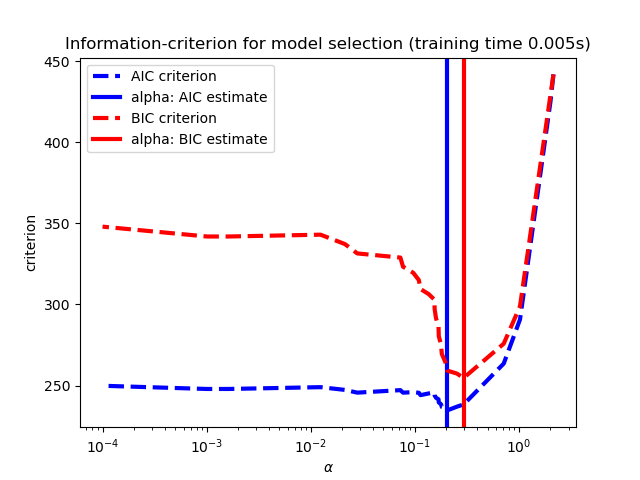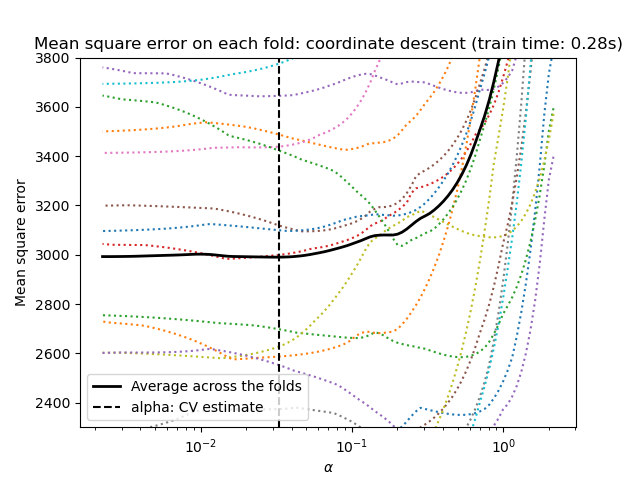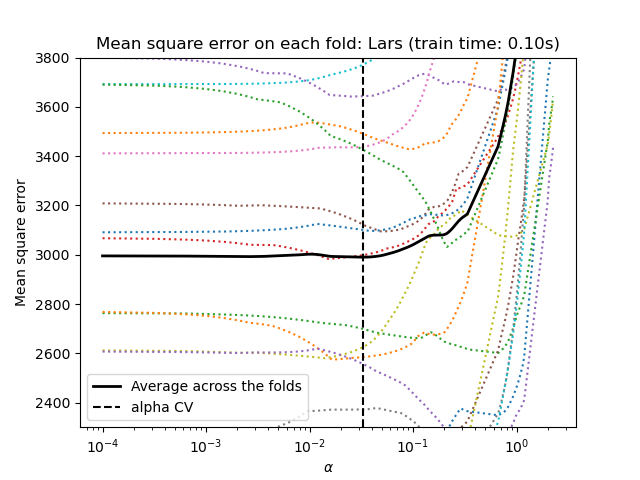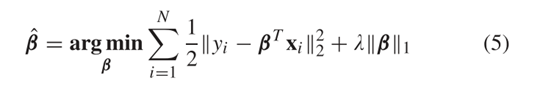• 文章目录线性回归引入重要参数重要属性重要方法例子岭回归引入重要参数重要属性重要方法示例Lasso 回归引入重要参数重要属性重要方法示例 本文主要讲一些sklearn中回归模型的使用，如果需要了解相关理论，请查阅：...
文章目录线性回归引入重要参数重要属性重要方法例子岭回归引入重要参数重要属性重要方法示例Lasso 回归引入重要参数重要属性重要方法示例
本文主要讲一些sklearn中回归模型的使用，如果需要了解相关理论，请查阅：【线性回归】面向新手的基础知识
线性回归
引入
from sklearn.linear_model import LinearRegression
# 默认参数如下：
LinearRegression(fit_intercept=True,normalize=False,copy_X=True,n_jobs=1)

重要参数
1，fit_intercept
bool类型，默认为True，表示是否计算截距(即 y = wx + b 中的 b )，推荐设置为True。
2，normalize
bool类型，默认为False，表示是否对各个特征进行标准化（默认方法是：减去均值并除以 $l_2$ 范数），推荐设置为True。如果设置为False，则建议在输入模型之前，手动进行标准化。
标准化的好处:

加速收敛

提升精度

注意：fit_intercept 设置为 False 时，将忽略此参数。

3，n_jobs
数值型，默认值为None。表示使用多少个处理器进行运算，当模型有多个回归目标( _targets > 1 )时，加速效果比较明显。推荐设置为 -1 ，此时会调用所有处理器。
重要属性
1，coef_
对应 X 各个特征的系数，绝对值越接近1，表示相关性越强。
2，intercept_
intercept_ 表示模型学习到的截距值。

注意：属性后面有下划线  _

重要方法
1，fit(X, y) ，训练模型
2，predict(X)，使用模型进行预测
3，score(X, y)，返回 $R^2$ 值 (确定系数) ，越接近1说明拟合的效果越好。
例子
import matplotlib.pyplot as plt
import numpy as np
from sklearn import datasets, linear_model
from sklearn.metrics import mean_squared_error, r2_score

# 载入糖尿病数据集

# 只从中抽取一个特征
diabetes_X = diabetes_X[:, np.newaxis, 2]

# 划分训练集与测试集
diabetes_X_train = diabetes_X[:-20]
diabetes_y_train = diabetes_y[:-20]
diabetes_X_test = diabetes_X[-20:]
diabetes_y_test = diabetes_y[-20:]

# 线性回归模型
regr = linear_model.LinearRegression(fit_intercept=True, normalize=True, n_jobs=-1)
regr.fit(diabetes_X_train, diabetes_y_train)
diabetes_y_pred = regr.predict(diabetes_X_test)
print('系数: \n', regr.coef_)

# 结果评估
print('均方误差: %.2f'
% mean_squared_error(diabetes_y_test, diabetes_y_pred))
print('确定系数(R^2): %.2f'
% r2_score(diabetes_y_test, diabetes_y_pred))

# 绘图
plt.scatter(diabetes_X_test, diabetes_y_test,  color='black')
plt.plot(diabetes_X_test, diabetes_y_pred, color='blue', linewidth=3)
plt.show()

输出结果：

系数:
[938.23786125]
均方误差: 2548.07
确定系数(R^2): 0.47岭回归
引入
# 加⼊L2正则化的线性回归
from sklearn.linear_model import Ridge
# 默认参数如下：
Ridge(alpha=1.0, fit_intercept=True, normalize=False, copy_X=True, max_iter=None, \
tol=0.001, solver='auto', random_state=None)

重要参数
1，alpha
正则项系数，初始值为1，数值越大，则对复杂模型的惩罚力度越大。
调参方法：

给定alpha较小的值，例如0.1。
根据验证集准确率以10倍为单位增大或者减小参数值。
在找到合适的数量级后，在此数量级上微调。

合适的候选值：[0.001, 0.01, 0.1, 1, 10, 100]
2，fit_intercept
bool类型，默认为True，表示是否计算截距(即 y = wx + b 中的 b )，推荐设置为True。
3，normalize
bool类型，默认为False，表示是否对各个特征进行标准化（默认方法是：减去均值并除以 $l_2$ 范数），推荐设置为True。如果设置为False，则建议在输入模型之前，手动进行标准化。
标准化的好处:

加速收敛

提升精度

注意：fit_intercept 设置为 False 时，将忽略此参数。

4，solver
求解优化问题的算法，推荐保持默认值’auto’，可以根据数据类型选择最合适的算法。可选的算法有：

‘svd’，采⽤用奇异值分解的⽅方法来计算
‘cholesky’，采⽤用scipy.linalg.solve函数求得闭式解。
‘sparse_cg’，采⽤用scipy.sparse.linalg.cg函数来求取最优解。
‘lsqr’，使用scipy.sparse.linalg.lsqr 求解，它是最快的。
‘sag’，使用随机平均梯度下降，当n_samples和n_features都较大时，通常比其他求解器更快。

5，max_iter
数值型，部分求解器需要通过迭代实现，这个参数指定了模型优化的最大迭代次数，推荐保持默认值None。
6，random_state
随机数种子，推荐设置一个任意整数，例如0，2020等，好处是模型可以复现。
7，n_jobs
数值型，默认值为None。表示使用多少个处理器进行运算，当模型有多个回归目标( _targets > 1 )时，加速效果比较明显。推荐设置为 -1 ，此时会调用所有处理器。
重要属性
1，coef_
对应 X 各个特征的系数，绝对值越接近1，表示相关性越强。
2，intercept_
intercept_ 表示模型学习到的截距值。

注意：属性后面有下划线  _

重要方法
1，fit(X, y) ，训练模型
2，predict(X)，使用模型进行预测
3，score(X, y)，返回 $R^2$ 值 (确定系数) ，越接近1说明拟合的效果越好。
示例
import matplotlib.pyplot as plt
import numpy as np
from sklearn import datasets, linear_model
from sklearn.metrics import mean_squared_error, r2_score

# 载入糖尿病数据集

# 划分训练集与测试集
diabetes_X_train = diabetes_X[:-20]
diabetes_y_train = diabetes_y[:-20]
diabetes_X_test = diabetes_X[-20:]
diabetes_y_test = diabetes_y[-20:]

# 岭回归模型
regr = linear_model.Ridge(alpha=0.001, fit_intercept=True, normalize=True, random_state=0)
regr.fit(diabetes_X_train, diabetes_y_train)
diabetes_y_pred = regr.predict(diabetes_X_test)
print('系数: \n', regr.coef_)

# 结果评估
print('均方误差: %.2f'
% mean_squared_error(diabetes_y_test, diabetes_y_pred))
print('确定系数(R^2): %.2f'
% r2_score(diabetes_y_test, diabetes_y_pred))

输出：

系数:
[   0.79171416 -236.95051097  511.18477367  327.22355517 -734.56024578
429.87194777   67.40320158  174.60152289  713.22832241   76.6722493 ]
均方误差: 2003.26
确定系数(R^2): 0.59

Lasso 回归
引入
from sklearn.linear_model import Lasso
Lasso(alpha=1.0, fit_intercept=True, normalize=False, precompute=False,
copy_X=True, max_iter=1000,tol=1e-4, warm_start=False,
positive=False,random_state=None,selection='cyclic')

重要参数
1，alpha
正则项系数，初始值为1，数值越大，则对复杂模型的惩罚力度越大。
调参方法：

给定alpha较小的值，例如0.1。
根据验证集准确率以10倍为单位增大或者减小参数值。
在找到合适的数量级后，在此数量级上微调。

合适的候选值：[0.001, 0.01, 0.1, 1, 10, 100]
2，fit_intercept
bool类型，默认为True，表示是否计算截距(即 y = wx + b 中的 b )，推荐设置为True。
3，normalize
bool类型，默认为False，表示是否对各个特征进行标准化（默认方法是：减去均值并除以 $l_2$ 范数），推荐设置为True。如果设置为False，则建议在输入模型之前，手动进行标准化。
标准化的好处:

加速收敛

提升精度

注意：fit_intercept 设置为 False 时，将忽略此参数。

4，precompute
bool 类型，默认值为False，决定是否提前计算Gram矩阵来加速计算。
5，max_iter
数值型，部分求解器需要通过迭代实现，这个参数指定了模型优化的最大迭代次数，推荐保持默认值None。
6，warm_start
bool类型，默认值为False。如果为True，那么使⽤用前⼀次训练结果继续训练。否则从头开始训练。
7，positive
bool类型，默认值为False。如果为True，那么强制要求权重向量的分量都为正数。
9，selection
字符串，默认值为"cyclic"。它指定了了当每轮迭代的时候，选择权重向量的哪个分量来更新：
(1) “random”: 更新的时候，随机选择权重向量的⼀个分量来更更新。
(2) “cyclic”: 更新的时候，从前向后依次选择权重向量的⼀个分量来更新。
10，random_state
随机数种子，推荐设置一个任意整数，例如0，2020等，好处是模型可以复现。
11，n_jobs
数值型，默认值为None。表示使用多少个处理器进行运算，当模型有多个回归目标( _targets > 1 )时，加速效果比较明显。推荐设置为 -1 ，此时会调用所有处理器。
重要属性
1，coef_
对应 X 各个特征的系数，绝对值越接近1，表示相关性越强。
2，intercept_
intercept_ 表示模型学习到的截距值。

注意：属性后面有下划线  _

重要方法
1，fit(X, y) ，训练模型
2，predict(X)，使用模型进行预测
3，score(X, y)，返回 $R^2$ 值 (确定系数) ，越接近1说明拟合的效果越好。
示例
import matplotlib.pyplot as plt
import numpy as np
from sklearn import datasets, linear_model
from sklearn.metrics import mean_squared_error, r2_score

# 载入糖尿病数据集

# 划分训练集与测试集
diabetes_X_train = diabetes_X[:-20]
diabetes_y_train = diabetes_y[:-20]
diabetes_X_test = diabetes_X[-20:]
diabetes_y_test = diabetes_y[-20:]

# LASSO回归模型
regr = linear_model.Lasso(alpha=0.08, fit_intercept=True, normalize=True, \
precompute=False,  copy_X=True, max_iter=1000,tol=1e-4, \
warm_start=False, positive=False, random_state=None,\
selection='cyclic')
regr.fit(diabetes_X_train, diabetes_y_train)
diabetes_y_pred = regr.predict(diabetes_X_test)
print('系数: \n', regr.coef_)

# 结果评估
print('均方误差: %.2f'
% mean_squared_error(diabetes_y_test, diabetes_y_pred))
print('确定系数(R^2): %.2f'
% r2_score(diabetes_y_test, diabetes_y_pred))

输出：

系数:
[   0.         -170.70972431  510.82478065  290.22047462  -71.2270991
-0.         -226.56455408    0.          480.30488212   54.40099876]
均方误差: 2004.60
确定系数(R^2): 0.59

可以看到有些系数为0，这也说明Lasso回归具有特征选择的作用。
参考文章：
sklearn.linear_model.LinearRegression
sklearn.linear_model.Ridge
sklearn.linear_model.Lasso
sklearn常用机器学习算法参数详解


展开全文机器学习 算法 python
• 利用Akaike信息准则（AIC）、Bayes信息准则（BIC）和交叉验证来选择Lasso估计量的正则化参数α的最优值。 LassoLarsIC获得的结果基于AIC/BIC标准。 基于信息准则的模型选择是非常快速的，但它依赖于对自由度的适当...
利用Akaike信息准则（AIC）、Bayes信息准则（BIC）和交叉验证来选择Lasso估计量的正则化参数α的最优值。
LassoLarsIC获得的结果基于AIC/BIC标准。
基于信息准则的模型选择是非常快速的，但它依赖于对自由度的适当估计，是针对大样本（渐近结果）导出的，并且假设模型是正确的，即数据实际上是由该模型生成的。当问题条件恶劣时（特征多于样本），它们也往往会中断。
对于交叉验证，我们使用两种算法计算套索路径：坐标下降（由LassoCV类实现）和Lars（最小角度回归）（由LassoLarsCV类实现）。两种算法得出的结果大致相同。它们在执行速度和数值误差来源方面有所不同。
Lars只为路径中的每个扭结计算路径解。因此，当只有很少的扭结时，它是非常有效的，如果特征或样本很少的话就是这种情况。无需设置任何参数，meta也能计算完整路径。相反，坐标下降计算预先指定网格上的路径点（这里我们使用默认值）。因此，如果网格点的数目小于路径中的扭结数目，则效率更高。如果特征的数量真的很大，并且有足够的样本来选择大量的特征，那么这样的策略可能会很有趣。在数值误差方面，对于高度相关的变量，Lars会累积更多的误差，而坐标下降算法只对网格上的路径进行采样。
注意α的最佳值是如何随每次折叠而变化的。这说明了当试图评估由交叉验证选择参数的方法的性能时，嵌套交叉验证是必要的：对于看不见的数据，这种参数选择可能不是最佳的。
import time

import numpy as np
import matplotlib.pyplot as plt

from sklearn.linear_model import LassoCV, LassoLarsCV, LassoLarsIC
from sklearn import datasets

# 这是为了避免在np.log10时被0除
EPSILON = 1e-4

X, y = datasets.load_diabetes(return_X_y=True)  # 导入数据

rng = np.random.RandomState(42)
X = np.c_[X, rng.randn(X.shape, 14)]  # 增加一些表现不好的特征

# 按Lars所做的标准化数据，以便进行比较
X /= np.sqrt(np.sum(X ** 2, axis=0))

# #############################################################################
# LassoLarsIC: 基于BIC/AIC准则的最小角度回归

model_bic = LassoLarsIC(criterion='bic')
t1 = time.time()
model_bic.fit(X, y)
t_bic = time.time() - t1  # 拟合模型运行时间
alpha_bic_ = model_bic.alpha_  # 打印alpha

model_aic = LassoLarsIC(criterion='aic')
model_aic.fit(X, y)
alpha_aic_ = model_aic.alpha_

# 画出所有alphas中信息标准（“aic”、“bic”）的值
def plot_ic_criterion(model, name, color):
criterion_ = model.criterion_  # 模型使用的标准
plt.semilogx(model.alphas_ + EPSILON, criterion_, '--', color=color,
linewidth=3, label='%s criterion' % name)
plt.axvline(model.alpha_ + EPSILON, color=color, linewidth=3,
label='alpha: %s estimate' % name)  # 标出最优alpha
plt.xlabel(r'$\alpha$')
plt.ylabel('criterion')

plt.figure()
plot_ic_criterion(model_aic, 'AIC', 'b')
plot_ic_criterion(model_bic, 'BIC', 'r')
plt.legend()
plt.title('Information-criterion for model selection (training time %.3fs)'
% t_bic)

# #############################################################################
# LassoCV: 坐标下降法

# Compute paths
print("Computing regularization path using the coordinate descent lasso...")
t1 = time.time()
model = LassoCV(cv=20).fit(X, y)
t_lasso_cv = time.time() - t1

# Display results
plt.figure()
ymin, ymax = 2300, 3800
plt.semilogx(model.alphas_ + EPSILON, model.mse_path_, ':')
plt.plot(model.alphas_ + EPSILON, model.mse_path_.mean(axis=-1), 'k',
label='Average across the folds', linewidth=2)
plt.axvline(model.alpha_ + EPSILON, linestyle='--', color='k',
label='alpha: CV estimate')

plt.legend()

plt.xlabel(r'$\alpha$')
plt.ylabel('Mean square error')
plt.title('Mean square error on each fold: coordinate descent '
'(train time: %.2fs)' % t_lasso_cv)
plt.axis('tight')
plt.ylim(ymin, ymax)

# #############################################################################
# LassoLarsCV: 最小角度回归

# Compute paths
print("Computing regularization path using the Lars lasso...")
t1 = time.time()
model = LassoLarsCV(cv=20).fit(X, y)
t_lasso_lars_cv = time.time() - t1

# Display results
plt.figure()
plt.semilogx(model.cv_alphas_ + EPSILON, model.mse_path_, ':')
plt.semilogx(model.cv_alphas_ + EPSILON, model.mse_path_.mean(axis=-1), 'k',
label='Average across the folds', linewidth=2)
plt.axvline(model.alpha_, linestyle='--', color='k',
label='alpha CV')
plt.legend()

plt.xlabel(r'$\alpha$')
plt.ylabel('Mean square error')
plt.title('Mean square error on each fold: Lars (train time: %.2fs)'
% t_lasso_lars_cv)
plt.axis('tight')
plt.ylim(ymin, ymax)

plt.show()展开全文算法
• 闲话Variable SelectionLasso 最近在看变量选择（也叫subset selection），然后来总结一下，想到哪写到哪的随意风格（手动微笑）。[11,12,13]是主要参考的综述文章。 Boosting 和 Stagewise Regression 嗯，我也...
原文：https://www.wengbi.com/thread_80323_1.html

闲话Variable Selection和Lasso

最近在看变量选择（也叫subset selection），然后来总结一下，想到哪写到哪的随意风格（手动微笑）。[11,12,13]是主要参考的综述文章。
Boosting 和 Stagewise Regression

嗯，我也很惊讶为什么这个Lasso会跟Boosting挂着勾。Lasso这样的带罚项的regression最早的思想来自于linear regression boosting，传统的boosting是只对残差进行boosting，然后对true function进行fitting，Incremental Forward Stagewise 则是依次选择跟残差最相关的特征（记得做归一化，血的教训带来的微笑），然后boosting，再某种条件下停止，达到选择特征同时对regression做shrinkage的目的。
与之很相似的是Forward Stepwiese Regression，一个是stepwise，一个是stagewise，stepwise在选择的时候依次直接加入，做fitting，而不是做residual boosting。但是Forward Stepwiese Regression是不稳定的，数据的微小改变会使变量的选择改变，之后选择的变量也会不同，最后选择的变量子集也会完全不同。一种变形是反向的Backward Stepwise Regression，先做全部变量的回归，然后依次拿掉变量。这两种算法都采用的贪婪策略，在每一步选择最优的改变，却无视特征的影响，所以不如stagewise。
Lasso

最常见的一种Lasso，最早在中提出，常见的正态分布残差假设的线性回归模型写作通过最小化残差平方和得到最优拟合，考虑L1的约束也可以用拉格朗日形式这样写出来的优化问题虽然是凸的，但不可导，无法直接求解，给定有限的样本，通常可以得到有偏的估计，也称作正则化的估计。
通常求解Lasso都是通过soft-thresholding，来进行一个阶段其中$\hat{\betaj^{\text{ls}}}$表示第$j$个变量的最小二乘估计，$(\cdot)+$表示取正数部分，与之相似的是加了L2正则的ridge regression的解为<ignore_js_op>闲话Variable Selection和Lasso-6.jpg (46.69 KB, 下载次数: 0)

下载附件

2018-11-17 11:36 上传

</ignore_js_op>

当回归的维数$p$大于样本数量$N$时，Lasso可以有效选择出有用的变量并且得到较好的回归效果。
自由度（df）对于调节参数$\lambda$的大小通常也很有用，常用的包括Akaike Information Criterion（AIC）和Bayesian Information Criterion（BIC），他们将模型的自由度也作为评判模型好坏的标准，AIC就定义为这里提一下，AIC和BIC标准定义如下：其中$\hat{L}$是最大似然函数，$n$是观测数据样本数量，$k$是模型中估计的参数，残差服从正态分布的时候就可以写成上面的形式。
尽管AIC关注于最小误差，关注BIC可以得到更加稀疏的模型。通常lasso估计的自由度可以用非零系数个数来表示，通过shrinking系数，来削减模型的自由度，这样我们可以选择一些变量同时丢弃一些变量，但要注意这只适用于$p <ignore_js_op>闲话Variable Selection和Lasso-9.jpg (66.44 KB, 下载次数: 0) 下载附件 2018-11-17 11:36 上传 </ignore_js_op> 对于正则项$\|\cdot\|^q_q$的选择，当$q\leq 1$的时候，可以达到变量缩减的目的。 目前Lasso的主流实现一般用二次规划，同时当KaTeX parse error: Expected 'EOF', got '&' at position 2: p&̲amp;gt;n的时候最多选择$n-1$个变量，这个由凸对偶性保证（只是看到，未经深究）。 lasso和robust regression lasso某种程度上也是一种鲁棒性回归，其中$Z$是一个disturbance的矩阵，$\theta$称为uncertainty set，lasso可以看做是有特殊不确定集的robust regression的求解。 LAR 最小角回归（Least Angle Regression）是今年比较流行的一种求解Lasso的方式，也是LAR出现之后，Lasso才被逐渐重视起来，主要思想是，经过归一化后，选择与$y$最相关的变量$\beta<i>j$加入（也就是加入第$j$个特征），然后增加$\beta</i>j$直至另一个变量比它俩更相关，重复此过程，直至没有变量与$y$相关或者函数足够拟合。 <ignore_js_op>闲话Variable Selection和Lasso-11.jpg (26.26 KB, 下载次数: 0) 下载附件 2018-11-17 11:36 上传 </ignore_js_op> 与之前的几种方式相比，LAR不用在第一步时候前进多步（boosting会有衰减$\epsilon$），而是直接到达合适位置直到第二个变量加入模型，也不用在多个变量之间迭代多步，而是在第三个变量加入模型前直接到达合适的点，下图就可以很好地说明 <ignore_js_op>闲话Variable Selection和Lasso-12.jpg (100.4 KB, 下载次数: 0) 下载附件 2018-11-17 11:36 上传 </ignore_js_op> Stepwise的方式是O-&gt;A-&gt;C，Stagewise的方式是O-&gt;B-&gt;E-&gt;C，注意其中O-&gt;B和B-&gt;E都是多步尝试后到达，LAR则是O-&gt;B-&gt;C，都是选择很合适的位置，其中BC平分角DBA，因为LAR选择时会在BA，BD同样相关的情况（LAR选择策略，一个step前进至两个变量和预测值具有相同相关性的位置）。以上都是在比较理想的情况，大部分高维的情况下都需要满足一定条件才会收敛。 LAR有几个比较remarkable的性质： 速度快，当$p &lt; n$时，需要$O(p^3+np^2)$次最小二乘拟合的计算可以用于拟合Lasso和stagewise模型可以使用简单的统计标准来选择步数，如$C_p$其中$k$是步数，$\hat{sigma}^2$是估计残差的方差，$C_p$提供了一种简单的停止算法的规则。 Pathwise coordinate descent for lasso [3, 4]中提到利用坐标下降的方式求解Lasso，求解lasso的原问题等价于求解其中$\hat{\beta}=\sumixiy_i$，是最小二乘系数，如果$\beta&gt;0时我们求偏导有此时我们就有soft-threshold的结果考虑用迭代的方式来依次计算每一个变量通过最小化每个变量多步迭代后收敛。这样相比于其他做lasso的方式而言，当变量个数增加时更加有高效，同时每个解都可以作为下一个变量求解的warm start。 Lasso的贝叶斯解释 我们可以将最小二乘解释为无参数分布先验时最大化后验概率估计的问题，Lasso可以视为Laplace先验或者是双指数分布先验，对于参数\beta$，Laplace先验为所以我们可以用Gibbs采样或者EM算法来做inference求解。 Lasso改进 任何形式的正则都会给估计值带来bias，同时很大程度降低了方差，另外，当真正的非零系数非常少，lasso会对正确的变量系数产生有偏估计。为了降低bias，中提出一种relaxed lasso，进行两阶段的估计，首先用lasso得到一些稀疏的变量，然后用低一些的正则系数来估计选择的变量，因此我们每一步都需要选择合适的regularization parameters，所以每步都需要进行cross-validation这样的方式来选择参数。 基于这种relaxed lasso，提出Variable Inclusion and Shrinkage Algorithm（VISA）的模型，在第二步中VISA并不忽略之前已经忽略的变量，而是给选择的变量更高的优先级。  提出一种adaptive lasso，给每个变量不同的重要性当$N\geq p$时，权重计算为当$N &lt; p$时，权重通过计算最小二乘估计时的最小正则项得到。中表明adaptive lasso在变量选择方面，比其他lasso模型更优。 里面提出elastic net，同时结合了L1和L2正则项除了在有correlated predictors上有较Lasso相比更好的表现，当$p&gt;N$的时候，Lasso最多只能选出$N$个预测值，但是elastic net可以选择超过$N$个预测值。当我们有group of relevant and redundant的变量时，Lasso通常只会从中选取一个并且忽略其他的，elastic net则会选择全部，同时平衡它们的参数估计值。 也有人考虑通过下采样的方式来增加稳定性和模型选择的准确度，所以称之为bolasso[9,10] Other Methods 除了上面提到的还有SCAD、Dantzig selector及其衍生算法等，并没有仔细研究过，就不误人子弟了。 后话 最近用到的大概是一个变量选择，我最开始考虑的是用RF做OOB，但是这次的特征有很多具有共线性，并没有找到RF对共线特征的表现，然后考虑lasso的方式，同时也关注这些模型对于共线性的鲁棒性，也是最近看到的ADL模型通常用到的，主要考虑了sklearn的lasso和lar，动手验证了一下，lar对于共线性的鲁棒性很好，相比之下lasso就差了很多，看了一下实现，发现lasso和ridge都是基于elastic net做coordinate descent，只不过设置$\alpha\$的值而已。
Reference
 Tibshirani, R. (1996). Regression Shrinkage and Selection via the Lasso. J. Roy. Stat. Soc. Ser. B, 58(1), 267–288.
 Efron, B., Johnstone, I., Hastie, T. &amp; Tibshirani, R. (2004). Least Angle Regression. Ann. Statist., 32(2), 407–499
 Friedman, Jerome, et al. &quot;Pathwise coordinate optimization.&quot; The Annals of Applied Statistics 1.2 (2007): 302-332.
 Wu, Tong Tong, and Kenneth Lange. &quot;Coordinate descent algorithms for lasso penalized regression.&quot; The Annals of Applied Statistics 2.1 (2008): 224-244.
 Meinshausen, N. (2007). Lasso with Relaxation. Comput. Stat. Data Anal., 52(1), 374–393.
 Radchenko, P. &amp; James, G. M. (2008). Variable Inclusion and Shrinkage Algorithms. J. Amer. Statist. Assoc.,
103(483), 1304–1315.
 Zou, H. (2006). The Adaptive Lasso and Its Oracle Properties. J. Amer. Statist. Assoc., 101(12), 1418–1429.
 Zou, H. &amp; Hastie, T. (2005). Regularization and Variable Selection via the Elastic Net. J. Roy. Stat. Soc. Ser. B, 67(2),
301–320
 Bach, F. R. (2008a). Bolasso: Model Consistent Lasso Estimation Through the Bootstrap. 15th International
Conference on Machine Learning; 33–40.
 Chatterjee, A. &amp; Lahiri, S. N. (2011). Bootstrapping Lasso Estimators. J. Amer. Statist. Assoc., 106(494), 608–625.
 Vidaurre, Diego, Concha Bielza, and Pedro Larrañaga. &quot;A survey of L1 regression.&quot; International Statistical Review 81.3 (2013): 361-387.
 Tibshirani, Robert. &quot;Regression shrinkage and selection via the lasso: a retrospective.&quot; Journal of the Royal Statistical Society: Series B (Statistical Methodology) 73.3 (2011): 273-282.
 Hesterberg, Tim, et al. &quot;Least angle and ℓ1 penalized regression: A review.&quot; Statistics Surveys 2 (2008): 61-93.
 Fan, Jianqing, and Runze Li. &quot;Variable selection via nonconcave penalized likelihood and its oracle properties.&quot; Journal of the American statistical Association 96.456 (2001): 1348-1360.
 Candes, Emmanuel, and Terence Tao. &quot;The Dantzig selector: Statistical estimation when p is much larger than n.&quot; The Annals of Statistics 35.6 (2007): 2313-2351.


展开全文• 原始套索算法：原lasso算法在普通最小二乘估计中引入了一个l1-范数惩罚项，如下所示，并使线性回归问题的解具有稀疏性，调整正则化参数λ以控制解的稀疏度。 本算法的创新点： 1、 用(负)对数似然函数替换最小二乘...
基于组套索的波段选择用于高光谱图像分类
贡献：
首先，是第一个介绍针对HSI的多标签土地覆被分类的频带选择问题的组套索算法的。其次，讨论了与HSIs特性相关的应用问题，并通过大量的实验和分析对性能进行了研究。
基础：
Lasso算法能够同时完成子集选择和线性回归的任务。
原始套索算法：原lasso算法在普通最小二乘估计中引入了一个l1-范数惩罚项，如下所示，并使线性回归问题的解具有稀疏性，调整正则化参数λ以控制解的稀疏度。本算法的创新点：
1、	用(负)对数似然函数替换最小二乘项外。GLBS算法被表述为(4)的(负)多项对数似然函数的极小化，并以系数矩阵矩阵的l1范数约束。所谓的(4)就是用来求解多项式分类问题的最大似然函数。
2、	在系数向量β的元素级别定义l1-范数约束。使用λ值来确保递增插入，随着λ值的减小，GLBS的基础套索属性可确保将频带递增地插入到最终分类模型中。稀疏罚项由整个矩阵的l1-范数变为系数行的l1-范数，保证了所实现的矩阵的行级稀疏性。βj代表某个光谱带j对所有K个土地覆盖类别的影响，因此β的行级稀疏性意味着将仅选择光谱带的一个子集，并且每个选定的带将有助于对所有光谱分类。
本文于其他文章不同的一个点：使用原始数据，不采取归一化
理由：尽管零均值和单位方差标准化可以帮助在严重吸收下扩大那些频率的信号差异，但它也将在整个频带上以固定的测量灵敏度来放大信号的噪声水平。 这将带来额外的噪音，从而导致分类准确性下降。 因此，最好不要经过标准化阶段而直接使用原始数据。
如有什么不正确的地方请指教，多多谅解！


展开全文• 文章目录Extended Comparisons of Best Subset Selection, Forward Stepwise Selection, and the Lasso摘要简介令人兴奋的新发展初步讨论 Extended Comparisons of Best Subset Selection, Forward Stepwise ...机器学习
• ## lasso

2017-12-01 21:38:39
Lasso(Least absolute shrinkage and selection operator, Tibshirani(1996))方法是一种压缩估计。它通过构造一个罚函数得到一个较为精炼的模型，使得它压缩一些系数，同时设定一些系数为零。因此保留了子集收缩的...
• 文章目录一、linear_model.LassoCV()♦ 正则化路径 regularization path♦ linear_model.LassoCV类① 自定义alpha范围测试② LassoCV默认参数配置测试 一、linear_model.LassoCV() 使用交叉验证的 Lasso类的参数看...机器学习
• 在这篇文章中，我们将首先看看Lasso和Ridge回归中一些常见的错误，然后我将描述我通常采取的步骤来优化超参数。代码是用Python编写的，我们主要依赖scikit-learn。本文章主要关注Lasso的例子，但其基本理论与Ridge...机器学习 数据挖掘 线性回归 ridge回归
• # use SelectFromModel meta-transformers along with Lasso to select the best couple of features from the Boston dataset import matplotlib.pyplot as plt import numpy as np from sklearn.datasets import ....sklearn
• 转自：https://blog.csdn.net/weixin_43374551/article/details/83688913 一、岭回归 1.参数推导 线性回归模型的目标函数J(β)=∑(y−Xβ)2J(β)=∑(y−Xβ)2J(β)=∑(y−Xβ)2J(β)=∑(y−Xβ)2J(β)=∑(y...机器学习 lambda
• lasso源自Least Absolute Selection and Shrinkage Operator，讲的是通过lasso回归选择了全部变量的一个子集，通过引入绝对值惩罚项来收缩系数。如果你只想知道题目，下面的内容其实可以不用...
• ## LASSO回归

千次阅读 2019-09-27 19:23:29
迭代算法，坐标轴下降法是沿着坐标轴下降，梯度下降是沿着梯度的负方向下降，对于p维参数的可微凸函数J(B)而言，如果存在一点B，使得函数J(B)在每个坐标轴上均达到最小值，则J(B)就是B上的全局最小值。 坐标...
• ## LASSO算法

千次阅读 2018-09-09 14:05:30
1.lasso族算法说明 在建立模型之初，为了尽量减小因缺少重要自变量而出现的模型偏差，通常会选择尽可能多的自变量。然而，建模过程需要寻找对因变量最具有强解释力的自变量集合，也就是通过自变量选择(指标选择、...
• ## Group Lasso

千次阅读 2020-02-24 16:15:26
Lasso（least absolute shrinkage and selection operator，又译最小绝对值收敛和选择算子、套索算法）是一种同时进行特征选择和正则化（数学）的回归分析方法，旨在增强统计模型的预测准确性和可解释性，最初由...
•  Lasso算法（least absolute shrinkage and selection operator，又译最小绝对值收敛和选择算子、套索算法）是一种同时进行特征选择和正则化（数学）的回归分析方法，旨在增强统计模型的预测准确性和可解释性，最初...
• ## Lasso学习

千次阅读 2016-10-13 21:07:21
Lasso的身世 Lasso的思想 Lasso的应用
• ## 回归问题-Lasso回归

万次阅读 多人点赞 2019-07-21 23:44:33
Lasso(Least absolute shrinkage and selection operator)方法是以缩小变量集（降阶）为思想的压缩估计方法。它通过构造一个惩罚函数，可以将变量的系数进行压缩并使某些回归系数变为0，进而达到变量选择的目的。 ...最小角回归 坐标下降法
• 在这篇文章中，我们将首先看看Lasso和Ridge回归中一些常见的错误，然后我将描述我通常采取的步骤来优化超参数。代码是用Python编写的，我们主要依赖scikit-learn。本文章主...python 机器学习 人工智能 深度学习 java
• 用最小角回归法求解Lasso回归的两个预备算法：前向选择（Forward Selection）算法，前向梯度（Forward Stagewise）算法 总结： Lasso回归是在ridge回归的基础上发展起来的。如果模型的特征非常多，需要压缩，...
• ## Lasso回归

万次阅读 多人点赞 2018-06-10 18:02:52
# Lasso 回归的参数 alpha = model.alpha_ lasso = Lasso(max_iter= 10000 , alpha=alpha) # 基于训练数据，得到的模型的测试结果,这里使用的是坐标轴下降算法（coordinate descent） y_pred_lasso = lasso.fit......